# EuroEconomica, Vol 35, No 2 (2016)

Stationarity of African Stock Markets under an ESTAR framework

Emmanuel Numapau Gyamfi1, Kwabena A Kyei2, Kwabena A Kyei3

Abstract: The paper investigates the stationarity of eight indices on eight African stock markets. We review the extant literature on the stationarity of African stock markets and build on the works of Zhang et al. (2012) and Smith and Dyakova (2014). We use the non-linear ADF unit root test and the modified Wald type test under an ESTAR framework in our study. Our results show that both non-linear unit root tests fail to reject the null of unit root in all the markets but for Botswana. We infer from our results that the stock markets in Egypt, Kenya, Mauritius, Morocco, Nigeria, South Africa and Tunisia are non-stationary and hence weak-form efficient. Our work goes to agree with Choi and Moh (2007) who believe that, the presence of non-linear pattern in a data has no effect on the performance of a unit root test if the non-linear process is far from a unit root process.

Keywords: Stationarity; African Stock Markets; ESTAR; Weak-form efficiency

JEL Classification: C12; G14

1. Introduction

The stationarity or otherwise of a stock market has implications on the conclusions that are made about such market(s) with respect to its informational eciency. The Ecient Market Hypothesis (EMH) as postulated by Fama (1970) states that, current prices reﬂect all the available information on the market. Thus information is quickly and instantaneously reﬂected in setting current security prices. This assertion implies a linear relationship between information and pricing. However, this linear assertion has been criticized by researchers such as Dyckman and Morse (1986) and Lee et al. (2001). They posit that the data generating process of a ﬁnancial data is not linear as the EMH stipulates. According to McMillan (2003), Hasanov and Omay (2008), the non-linearity of a ﬁnancial data arises because of issues such as market friction, transaction costs, noise traders, the existence of bid-ask spreads and short sales. The issues raised by these researchers make the EMH assertion of linearity not totally valid. Notwithstanding these issues raised, there have been studies of the EMH on African stock markets especially the weak-form type of EMH. The weak-form EMH states that prices on the market reﬂect information on past prices. Studies of the weak-form EMH on African stock markets have ended with mixed conclusions. The mixed conclusions on African markets were summarized by Afego (2015). An example of a mixed conclusion about the markets was when Jeeris and Smith (2005) tested for weak-form eciency in South Africa, Zimbabwe, Nigeria, Morocco, Kenya, Egypt and Mauritius by a GARCH model with time varying parameters. They found the JSE to be ecient over the study period between 1990 and 2001, Nigeria, Egypt and Morocco only became ecient in the latter part of the period, while Zimbabwe and Kenya failed the eciency test over the whole period. However, Smith (2008) studied the weak-form EMH on 11 African markets SA, Zimbabwe, Ghana, Nigeria, Egypt, Tunisia, Botswana, Kenya, Morocco, Mauritius and Cote d’Ivoire. Smith who analyzed the data using Wrights joint variance ratio test, proposed by Wright (2000), and Chow-Denning multiple variance ratio test, proposed by Chow and Denning (1993) observed that none of the markets studied was weak form ecient. There are so many questions that come to mind as to why this contradiction. Could it be of the data period chosen or the method of analysis employed?

This study seeks to use a non-linear method to analyze the stationarity or otherwise of eight African stock markets since according to Afego (2015), the methods that have been used in the literature in analyzing data on African stock markets have been linear. Linear models in analyzing stationarity have been biased towards the rejection of a unit root when applied to data with non-linear pattern. Hence we consider non-linear time series models such as the smooth transition autoregressive (STAR) models which have been used by researchers for some time now in analyzing ﬁnancial data, Terasvirta (1994). We first test for linearity in the series by applying the Brock, Dechert and Scheinkman (1987) test for detecting serial dependence in time series. After the evidence of nonlinearity in the series, we proceeded in testing for stationarity by employing two non-linear unit root tests under an exponential STAR (ESTAR) framework; the ADF type unit root against ESTAR developed by Kapetanios et al. (2003) and the modiﬁed Wald type non-linear unit root test by Kruse (2011).

The rest of the paper is organised as follows:

Section two describes the data and the methods used in analyzing the data. The results and ﬁndings are presented in section three. Section four discusses the ﬁndings and section ﬁve concludes the paper.

2. Data and Methodology

We considered eight indices from eight African countries. The data obtained from DATASTREAM was from 28 August 2000 to 28 August 2015. We used the logarithmic daily closing prices of the indices for our analysis.

Table 1 presents the countries and their respective indices.

Table 1. Representative Indices of Countries

 Country Representative Index Botswana S&P Botswana BMI Egypt EGX 30 Kenya NSE 20 Mauritius SE Semdex Morocco Morocco All Share (MASI) Nigeria Nigeria All Share South Africa FTSE JSE All Share Tunisia Tunindex

BDS Linearity Test

The BDS test was developed by W.A. Brock, W. Dechert and J. Scheinkman in 1987 (hereafter BDS (1987)). The test is used for detecting serial dependence in time series, hence it can be used to detect nonlinearity. The null of independent and identically distributed (I.I.D.) hypothesis is tested against an unspecified alternative. We follow the procedure below in computing the BDS test:

Let 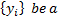time series with N observations, which should be the first difference of the natural logarithms of raw data in time series. Thus

Equation 1

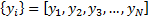

We select a value of m called the embedding dimension and embed the time series into m-dimensional vectors, by taking each m successive points in the series. This converts the series of scalars into a series of vectors with overlapping entries.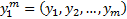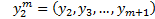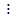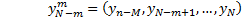We compute the correlation integral, which is a measure of the spatial correlation among the points, by adding the number of pairs of points 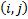 where

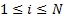and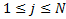, in the m-dimensional space which are “close” in the sense that the points are within a radius or tolerance  of each other.

Equation 2

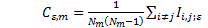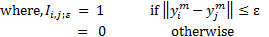BDS (1987) proved that if the time series is I.I.D

Equation 3

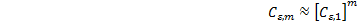

According to Lin (1997), if the ratio 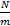 is greater than 200, the values of  range from 0.5 to 2 and the values of m are between 2 and 5 (Brock et al., 1988).

The quantity 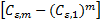has an asymptotic normal distribution with zero mean and a variance 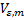 defined as:

Equation 4

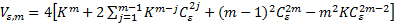

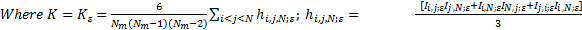

The BDS test statistic is thus stated as:

Equation 5

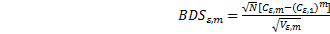

We reject the null of independent and identically distributed (I.I.D.) at the 5% significance level if 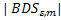 > 1.96.

The KSS Test

The Augmented Dickey-Fuller (ADF) test which is to detect nonstationarity in a time series was extended by Kapetanios, Shin and Snell (2003) (hereafter, the KSS). The null of a unit as in the ADF test is kept by KSS but the alternative hypothesis is that of a nonlinear but globally stationary process. We explain the KSS test as follows:

Let

Equation 6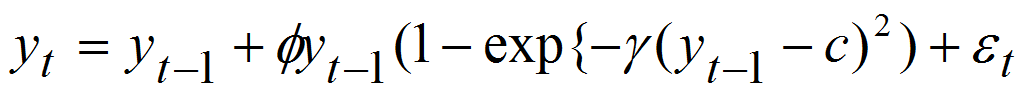be a univariate exponential smooth transition autoregressive model of order 1, ESTAR (1) where c is the location parameter.

Equation (6) was transformed by KSS (2003) by making c=0. Hence we obtained:

Equation 7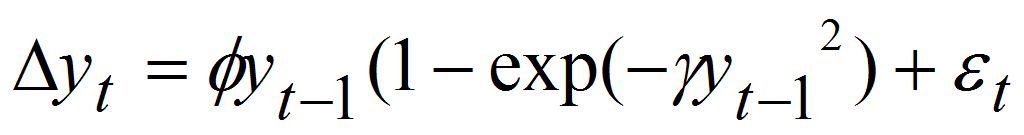Where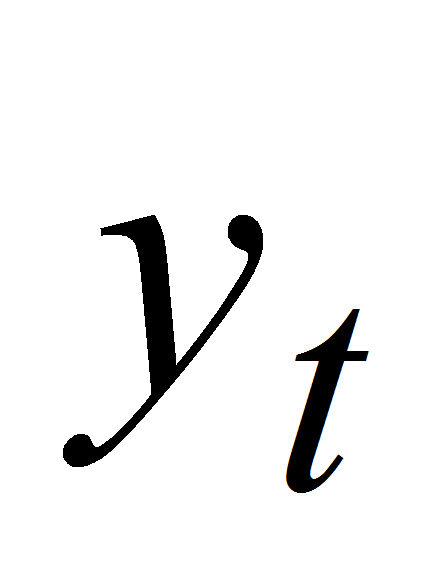is the demeaned and or detrended series of interest and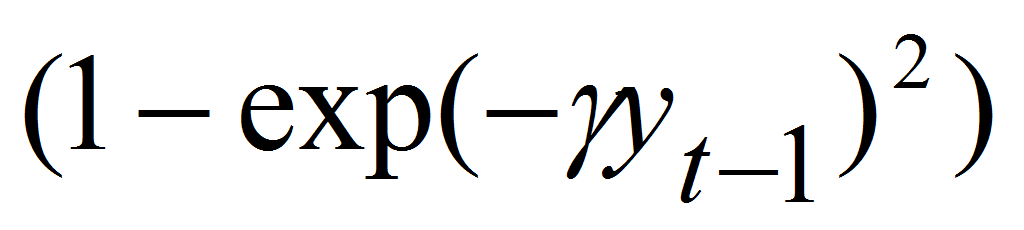is

the exponential transition function in the KSS test for nonlinear adjustment. The hypotheses of the KSS test is that Ho: γ = 0 against Ho: γ > 0. Sincecannot be identified directly under Ho, the null hypothesis becomes impossible to test. KSS (2003) therefore reparametrized Equation (7) using a first order Taylor series approximation and the following auxiliary regression was obtained:

Equation 8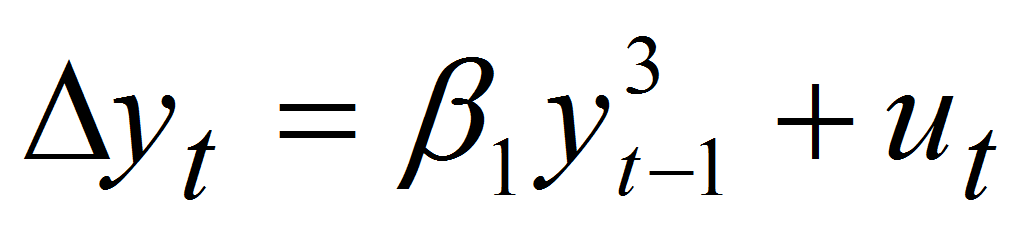With ut being a noise term depending on εt, 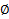 and remainder of the Taylor expansion. Equation (8) looks like the Dickey – Fuller test regression without deterministic term. The cubic term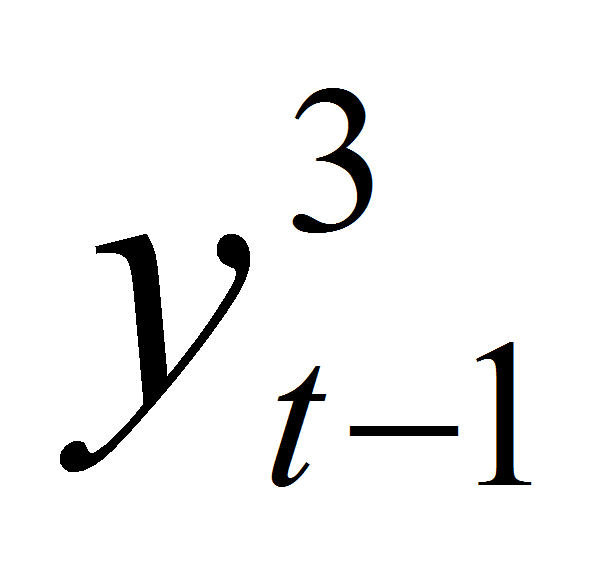approximates the ESTAR nonlinearity.

We modify Equation (8) so that it can take care of the presence of a possible serial correlation in the error terms. Thus

Equation 9+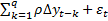

Where q is the number of augmentations that can be specified using any standard lag length selection criteria. Hence the null becomes Ho : 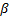1 = 0 versus H1: 1 < 0.

KSS (2003) provided critical values for the test statistic which had no asymptotic standard normal distribution. The critical values on page 364 of their article are reproduced in Table 2.

Table 2. Critical Values of the KSS test

 Significance level Raw Data Demeaned Data Detrended Data 1% -2.82 -3.48 -3.93 5% -2.22 -2.93 -3.40 10% -1.92 -2.66 -3.13

Kruse (2011) Modified Wald Type test

The argument by Kruse (2011) was that the location parameter c in the exponential transition function must be nonzero hence the nonlinear model in Equation (6) was considered as:

Equation 10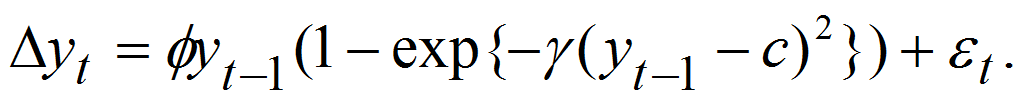We apply a first-order Taylor approximation to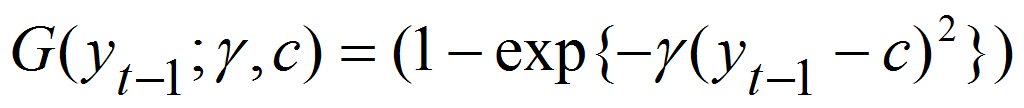around γ = 0 and proceed with the test regression as follows:

Equation 11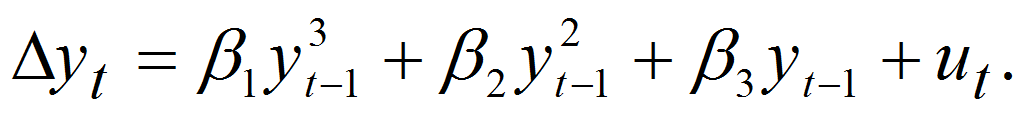Following Kapetanions et al. (2003), and to improve the power of the test statistic, we impose 3 = 0 so we obtain an estimated model as:

Equation 12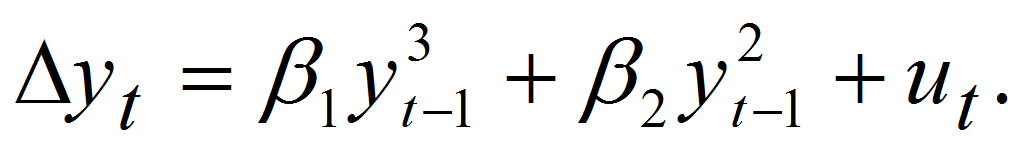where 1 = γand 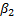 = -2c γ. Our interest is in testing the null of a unit root

Ho: γ = 0 against the alternative of a globally stationary ESTAR process H1: γ > 0

From the test regression in Equation (11), this pair of hypothesis is equivalent to Ho: 1 = 2 = 0 against H1 : 1 < 0, 2 ≠ 0. It is to be noted that under H1, 1 is one-sided and 2 is two-sided so that c is allowed to take on real values. This testing problem where one parameter is one sided under H1 and the other two sided is non – standard hence employing a standard Wald test would be inappropriate. Following the methods of Abadir and Distaso (2007) Kruse (2011) derived a modified Wald test for the null and alternative hypothesis which is simply formulated by:

Equation 13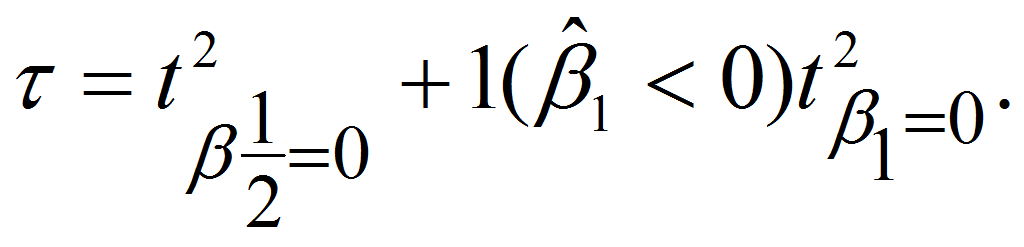Where two-summands in the test statistic 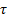can be interpreted as follows: the first term is a squared t – statistic for the hypothesis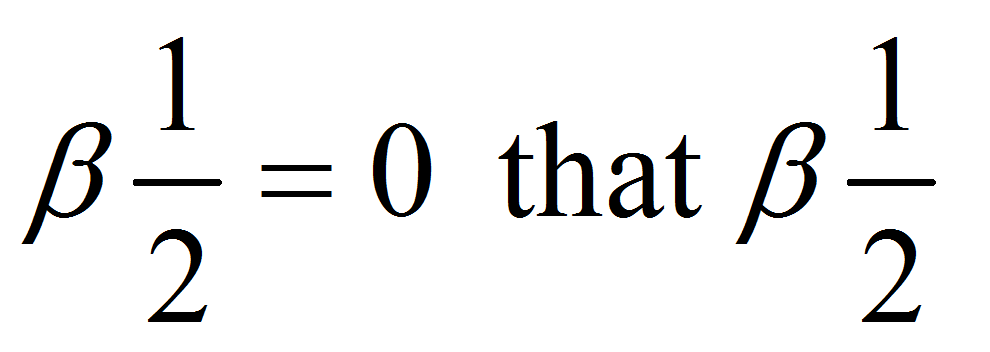is orthogonal to 1 while the second term is a squared t-statistic for the hypothesis 1 = 0.

The test statistichas a non-standard asymptotic distribution and the asymptotic critical values are derived under the standard assumptions for the error term which are shown in Table 3.

Table 3. Critical values of the Kruse test

 Significance level Raw Data Demeaned Data Detrended Data 1% 13.15 13.75 17.10 5% 9.53 10.17 12.82 10% 7.85 8.60 11.10

3. Results and Findings

The linear unit root test results in Table 4 shows that the ADF and KPSS tests fail to reject the assertion that the series is of unit root in all cases. This means that the logarithmic daily closing prices of the indices under study are non-stationary and therefore not mean reverting.

Table 4. Results of Linear tests

 Index ADF KPSS S&P Botswana BMI -1.55 22.51 EGX 30 -0.82 17.78 NSE 20 -1.23 15.42 SE Semdex -0.74 23.80 Morocco All Share (MASI) -0.51 16.61 Nigeria All Share -1.67 12.25 FTSE/JSE All Share -1.98 24.37 Tunindex -1.84 25.17

We investigated whether the logarithmic daily closing prices of the indices were characterized by a linear or nonlinear trend. We employed the Brock, Dechert and Scheinkman (BDS) (1996) test for detecting serial dependence in time series. The results of the BDS test of embedding dimension (m = 2) and metric bound (ε) equals 0.5 times the standard deviation of the logarithmic price series as shown in Table 5 indicate that all the test statistics are greater than the critical values. Also, the small p-values show that a non-linear relationship exists. Therefore our conclusion on the BDS test is that the null of an independent and identically distribution is rejected. This shows that the eight indices follow a non-linear pattern.

Table 5. BDS test results

 S&P Botswana EGX 30 NSE 20 SE Semdex MASI Nigeria All Share FTSE/JSE All Share Tunindex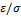0.5 0.5 0.5 0.5 0.5 0.5 0.5 0.5 m 2 2 2 2 2 2 2 2 Test statistic 192.74 178.25 150.04 182.84 176.96 182.21 238.74 247.50 P-value 0.00 0.00 0.00 0.00 0.00 0.00 0.00 0.00

This non-linear pattern in the data therefore allowed us to carry out a non-linear test on the data using the non-linear ADF test of Kapetanios et al. (2003) and the modiﬁed Wald type test of Kruse (2011). However, plots of the logarithmic daily closing prices is characterized by a trend, hence our non-linear analysis will only be on the demeaned and detrended data.

The results obtained by analyzing the data using the non-linear Kapetanios et al. (2003) and the non-linear Kruse (2011) tests as shown in Tables 6 and 7 respectively fail to reject the null of unit root in all the markets studied but for Botswana in only one case. The Kapetanios et al. (2003) test rejects the null of unit root for Botswana when the data is demeaned at the 5% and 10% signiﬁcance levels while the Kruse (2011) test rejects the null of unit root for Botswana when the data is demeaned at the 1%, 5% and 10% signiﬁcance levels.

Table 6. Results of Kapetanios et al. (2003) test

 S&P Botswana EGX 30 NSE 20 SE Semdex MASI Nigeria All Share FTSE/JSE All Share Tunindex Demeaned -3.15 -0.94 -0.77 -1.08 -1.27 -1.92 -1.03 -0.31 Detrended -1.28 -1.15 -1.18 -1.03 -1.16 -0.95 -2.06 -2.01

Table 7. Results of Kruse (2011) test

 S&P Botswana EGX 30 NSE 20 SE Semdex MASI Nigeria All Share FTSE/JSE All Share Tunindex Demeaned 18.59 1.04 1.02 1.55 1.97 4.31 2.47 1.24 Detrended 5.95 1.43 1.57 1.81 1.36 1.66 7.19 4.17

4. Discussion

The non-stationarity conclusion and the subsequent implication of weak-form eciency of seven out of the eight African markets studied contradicts most studies in the weak-form eciency literature of the EMH. In most of the studies summarized by Afego (2015), with the exception of the South African market which has shown consistency in being weak-form ecient, the rest of the markets have been weak-form inecient. The reason behind the results of this study which concludes weak-form eciency for the markets known in literature to be mostly weak-form inecient might be due to the assertion by Cajueiro and Tabak (2004b).They are of the view that markets become ecient through time. The markets studied have existed for quite some time. As a result, factors given by Cajueiro and Tabak (2004b) to bring about eciency such as increases in foreign capital inﬂow and in trading volumes, technological advancement in a market’s operations might have aected our conclusion.

On the other hand, Botswana was proven to be weak-form inecient. The reason for this might be due to the fact that even though the market had existed since 1989, technological advancement in the market has been slow. For example, automated trading system which deals with clearing and settlement was recently introduced in 2012.

It is to be noted that, though the BDS test showed non-linear pattern in the eight indices hence the need to analyze the series using non-linear models, there are no significant differences in the results and conclusions obtained from analyzing the data using both the linear ADF and KPSS unit root tests and the non-linear ADF and the modified WALD test. This observation goes to agree with Choi and Moh (2007) who are of the belief that, the presence of non-linear pattern in a data has no effect on the performance of a unit root test if the non-linear process is far from a unit root process.

5. Conclusion

We investigated the stationarity of eight indices on eight African stock markets. The BDS test of serial dependence was used to check whether the data generating process was characterized by a linear or non-linear pattern. The BDS test results showed non-linearly behaviour in the data generating process hence we employed two non-linear unit root tests under the ESTAR framework namely the non-linear ADF unit root of Kapetanios et al. (2003) and an extension of the Kapetanios et al. (2003) test known as the modiﬁed Wald type test of Kruse (2011).

Our ﬁndings from the two non-linear tests show that the markets are non-stationary but for Botswana, a conclusion not significantly different from the results obtained using the linear unit root tests.

Thus we conclude the stock markets in Egypt, Kenya, Mauritius, Morocco, Nigeria, South Africa and Tunisia are non-stationary therefore weak-form ecient whiles Botswana is not weak-form ecient. This observation means it is dicult for arbitrageurs to make abnormal proﬁts by analyzing the price history on these seven African stock markets.

6. References

Abadir, K.M. & Distaso, W. (2007). Testing joint hypotheses when one of the alternatives is one-sided. Journal of Econometrics, 140, pp. 695-718.

Afego, P. (2015). Market Eciency in Developing African Stock Markets: What do we know?. The Journal of Developing Areas, 49(1), pp. 243-266.

Brock, W.A.; Dechert, W. & Scheinkman, J. (1987). A test of independence based on the correlation dimension. Econometric Review, 15, pp. 197 – 235.

Cajueiro, D. & Tabak, B. (2004b). The Hurst exponent over time: testing the assertion that emerging markets are becoming more ecient Phys. A Stat. Theor. Phys., 336 (3), pp. 521-537.

Choi, C.Y & Moh, Y.K. (2007). How useful are tests for unit-root in distinguishing unit-root processes from stationary but non-linear processes? Econometrics Journal, 10, pp. 82–112.

Chow, K.V. & Denning, K.C. (1993). A simple multiple variance ratio test. Journal of Econometrics, 58(3), pp. 385-401.

Dyckman, Thomas R. & Dale, Morse. (1986). Ecient Capital Markets and Accounting: A Critical Analysis. Prentice-Hall.

Fama, E.F. (1970). Ecient Capital markets: A Review of theory and empirical work. Journal of Finance, 25(2), pp. 383-417.

Hasanov, M. & Omay, T. (2008). Nonlinearities in emerging stock markets: evidence from Europe’s two largest emerging markets. Appl. Econ. 40, pp. 2645-2658.

Jefferis, K. & Smith, G. (2005). The Changing Eciency of African Stock Markets. South African Journal of Economics, 73, pp. 54–67.

Kapetanios, G.; Shin, Y. & Snell, A. (2003). Testing for a unit root in the nonlinear STAR framework. J. Econ., 12, pp. 359–379.

Kruse, R. (2011). A new unit root test against ESTAR based on a class of modiﬁed statistics. Stat. Pap, 52, pp. 71-85.

Lee, C.F.; Chen, G.M. & Rui, O.M. (2001). Stock returns and volatility on Chinas stock markets. Journal of Financial Research, 24(4), pp. 523-543.

McMillan, D.G. (2003). Non-linear predictability of UK stock market returns. Oxf. Bull. Econ. Stat., 65, pp. 557-573.

Smith, G. & Dyakova, A. (2014). African Stock Markets: Efficiency and Relative Predictability. South African Journal of Economics, 82(2), pp. 258-275.

Smith, G. (2008). Liquidity and the Informational Eciency of African Stock Markets. South African Journal of Economics, 76(2), pp. 161-175.

Terasvirta, T. (1994). Specification, estimation, and evaluation of smooth transition autoregressive models. Journal of the American Statistical Association, 89, pp. 231-240.

Wright, J.H. (2000). Alternative variance-ratio tests using ranks and signs. Journal of Business and Economic Statistics, 18(1), pp. 1-9.

Zhang et. al (2012). Revisiting the Efficient Market Hypothesis for African countries: Panel SURKSS test with a Fourier function. South African Journal of Economics, 80(3), pp. 2287-300.

1 Department of Statistics, University of Venda, South Africa, winterblaze1707@gmail.com.

2 Department of Statistics, University of Venda, South Africa, kyei@univen.ac.za.

3 Department of Mathematics, University of Louisville, USA, ryan.gill@louisville.edu.

### Refbacks

• There are currently no refbacks.